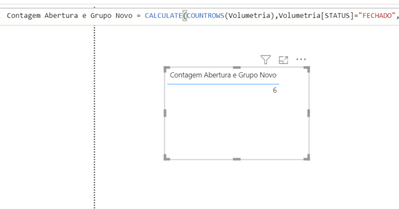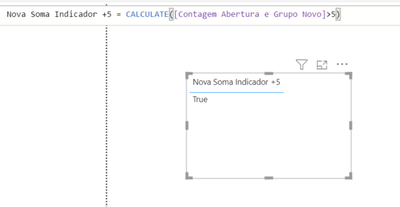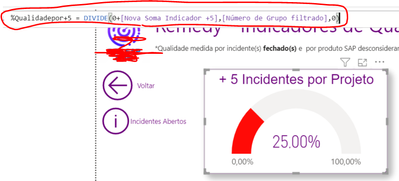cancel
Showing results for
Did you mean:New Member

## Calculate rows of distinct groups that have more than "5 requests"

Hello dear.
I have a doubt in calculating the number of lines for Groups that have more than 5 Requests. In this case, I have an indicator that the total base is the number of distinct groups and of which there are more than 5 requests.
Example:

Group A: 2 Requests
Group B: 7 Requests
Group C: 6 Requests
Group 😧 1 Request

So, there are 4 groups and only two of them have more than 5 requests (>5).

So my indicator (speedometer) should show 50%.

---------------------------------------------------------------------------------------------------------

So far these are the calculations and commands I created, but they are not working:

1.) I did the calculation first to count the lines with a filter:----------------------------------------------------------------------------------------------------------

2.) Then I calculated this row calculation to show which row has more than 5

- I think this is the problem with the calculation---------------------------------------------------------------------------------------------

3.) Here's just the distinct groups I need, that's right---------------------------------------------------------------------------------------------

Next is shown in the 25% indicator, it is incorrect. It should show 0%, since none of the groups presented more than 5 requests:Could you help me with this calculation?

Thanks

1 ACCEPTED SOLUTIONResolver I

Hi, @matiellu2

Try the codes below:

``Count = DISTINCTCOUNT('Table'[Status])``
``````Count>5 =
var tab=SUMMARIZE('Table','Table'[Status],"Count",COUNTROWS('Table'))
return CALCULATE(DISTINCTCOUNT('Table'[Status]),FILTER(tab,[Count]>5))``````
``Percentage = [Count>5]/[Count] ``Sample pbix

Regards,

EthanResolver I

Hi, @matiellu2

Try the codes below:

``Count = DISTINCTCOUNT('Table'[Status])``
``````Count>5 =
var tab=SUMMARIZE('Table','Table'[Status],"Count",COUNTROWS('Table'))
return CALCULATE(DISTINCTCOUNT('Table'[Status]),FILTER(tab,[Count]>5))``````
``Percentage = [Count>5]/[Count] ``Sample pbix

Regards,

Ethan